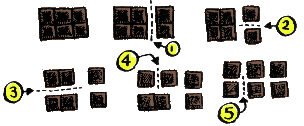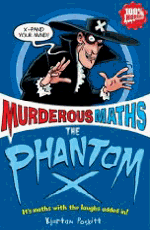AGE BY CHOCOLATE!

There's been an email trick going round that works like this:

 First of all, pick the number of times a week that you would like to have chocolate (more than once but less than 10) Let's assume it's 7 Multiply this number by 2 7 x 2 = 14. Add 5 14 + 5 = 19Multiply it by 50. You might need the chocolate calculator! 19 x 50 = 950 If you have already had your birthday this year add 1770*. If you haven't, add 1769* * These numbers make the trick work in the year 2020. In 2021, use 1771 if you've had your birthday or 1770 if not. In 2022, use 1772 if you've had your birthday or 1771 if not. Let's say it's the year 2020 and we've had a birthday so we add 1770 +950 to get 2720. Now subtract the four digit year that you were born. Let's say we were born in 1995, so 2720 - 1995 = 725 You should end up with a three digit number. The first digit is the number of times you like chocolate in the week. The last two digits are your age! Yes! 7 is the number picked and if we were born in 1995, then in 2020 we would be 25 years old!

So what's going on?This is a variation on the old "Think of a Number" trick which can be explained with algebra.

First of all, when we look at the answer 725, we know the 7 is the number we chose for chocolate. The trick makes sure that this number is multiplied by 100 so that it appears in the "hundreds" place in the answer.

As for the age, all the trick does is subtract your year of birth from this year, but it does it in a disguised way. Instead of just subtracting from 2020, it first adds 250 + 1770 to get 2020 before you subtract your birth year.

Confused? Never mind, let's do it again with algebra...

 How many snaps does it take to break up a chocolate bar? You might find this puzzle tastier than the algebra!It takes 5 snaps to break up this little bar into the six squares. (You're not allowed to break two pieces at the same time.)These bars both have 12 squares. Which will need the most snaps? Or will they both need the same number? You can find the answer on this page: Easy Questions Evil AnswersYou'll find a lot more great tricks and how they all work in The Phantom X